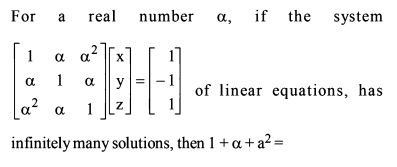# Basic Maths Grand Test- Q22asked Nov 19
reshown Nov 20

it is given  but i suppose it is

So now to solve the linear equations let us first have our augmented matrix

applying  and

again applying

Now that we have got here we see that in question it is given that this linear equations have infinitely many solutions so the value of  must be zero otherwise we would get 3 independent rows that would imply that the matrix is invertible and we will be left with only one solution for any right hand side.

as we make  as zero also we see that  also be zero as it will make the equations consistent

from above we conclude that the value of  = -1.

So by putting the value in rthe equation  we get the answer as 1.answered Nov 24 by (1,040 points)